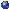## Events

##### Groups And DynamicsNathanael Berestycki, zoom: Cutoff phenomenon for random walks on random graphs
Thursday, February 11, 2021, 12:30pm - 01:30pm
Let $G = G(n, \lambda / n)$ be an instance of the Erdos-Renyi random graph model on $n$ vertices, with edges present independently of one another with probability $p = \lambda / n$. When $\lambda1$, it is known that this random graph has a unique giant component with high probability as $n \to \infty$ (where giant means that it contains a positive fraction of all vertices). What can we say about the mixing time of random walk on this giant component? It turns out that the answer depends very much on the starting point of the walk. In this work we show that from a typical starting point the cutoff phenomenon takes place. The limiting order of the mixing time depends on the speed and the entropy of random walk on a Galton-Watson tree which is the Benjamini-Schramm limit of the giant component of a random graph. The result extends to graphs with prescribed degree sequences.Location: zoom